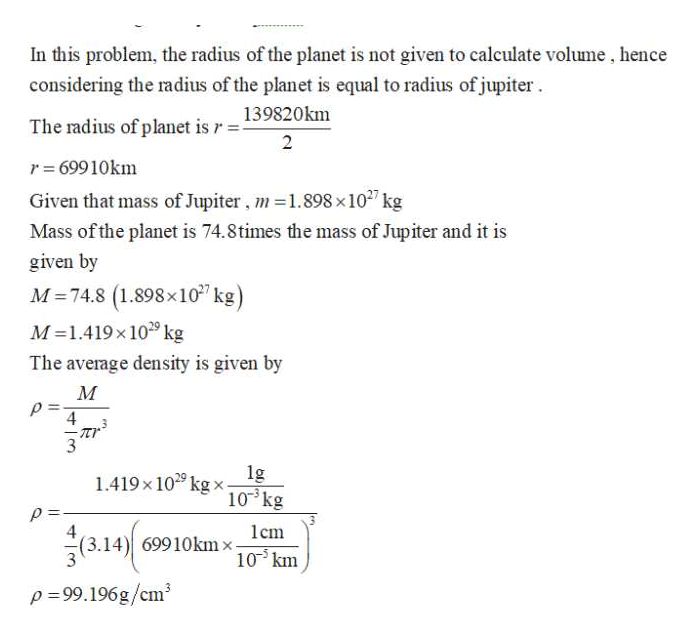# The mass of the planet is approximately 74.8 times the mass of Jupiter. Calculate the average density of the planet. Give your answer in grams per cubic centimeter.

Question
10 views

The mass of the planet is approximately 74.8 times the mass of Jupiter. Calculate the average density of the planet. Give your answer in grams per cubic centimeter.

check_circle

Step 1

Write the average densit...help_outlineImage TranscriptioncloseIn this problem, the radius of the planet is not given to calculate volume, hence considering the radius of the planet is equal to radius of jupiter 139820km The radius of planet is r- 2 r 69910km Given that mass of Jupiter, m1.898 x 1027 kg Mass ofthe planet is 74.8times the mass of Jupiter and it is given by M 74.8 (1.898x10 kg) M 1.419x109 kg The average density is given by М 4 1.419x10 kg x 10 kg 4 (3.14) 69910kmx lcm 10km p-99.196g/cm3 fullscreen

### Want to see the full answer?

See Solution

#### Want to see this answer and more?

Solutions are written by subject experts who are available 24/7. Questions are typically answered within 1 hour.*

See Solution
*Response times may vary by subject and question.
Tagged in

### Physics